Open In App
Related Articles
• Write an Interview Experience
• Discrete Mathematics Tutorial

# Prim’s Algorithm for Minimum Spanning Tree (MST)

## Introduction to Prim’s algorithm:

We have discussed Kruskal’s algorithm for Minimum Spanning Tree. Like Kruskal’s algorithm, Prim’s algorithm is also a Greedy algorithm. This algorithm always starts with a single node and moves through several adjacent nodes, in order to explore all of the connected edges along the way.

The algorithm starts with an empty spanning tree. The idea is to maintain two sets of vertices. The first set contains the vertices already included in the MST, and the other set contains the vertices not yet included. At every step, it considers all the edges that connect the two sets and picks the minimum weight edge from these edges. After picking the edge, it moves the other endpoint of the edge to the set containing MST.

A group of edges that connects two sets of vertices in a graph is called cut in graph theory. So, at every step of Prim’s algorithm, find a cut, pick the minimum weight edge from the cut, and include this vertex in MST Set (the set that contains already included vertices).

## How does Prim’s Algorithm Work?

The working of Prim’s algorithm can be described by using the following steps:

Step 1: Determine an arbitrary vertex as the starting vertex of the MST.
Step 2: Follow steps 3 to 5 till there are vertices that are not included in the MST (known as fringe vertex).
Step 3: Find edges connecting any tree vertex with the fringe vertices.
Step 4: Find the minimum among these edges.
Step 5: Add the chosen edge to the MST if it does not form any cycle.
Step 6: Return the MST and exit

Note: For determining a cycle, we can divide the vertices into two sets [one set contains the vertices included in MST and the other contains the fringe vertices.]

Recommended Practice

## Illustration of Prim’s Algorithm:

Consider the following graph as an example for which we need to find the Minimum Spanning Tree (MST).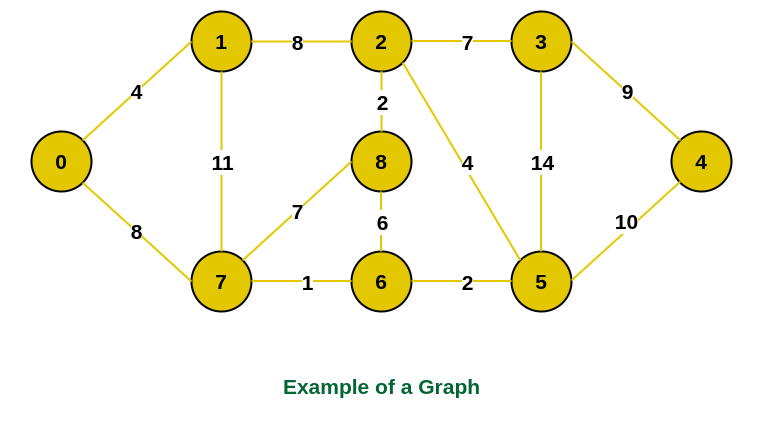Example of a graph

Step 1: Firstly, we select an arbitrary vertex that acts as the starting vertex of the Minimum Spanning Tree. Here we have selected vertex 0 as the starting vertex.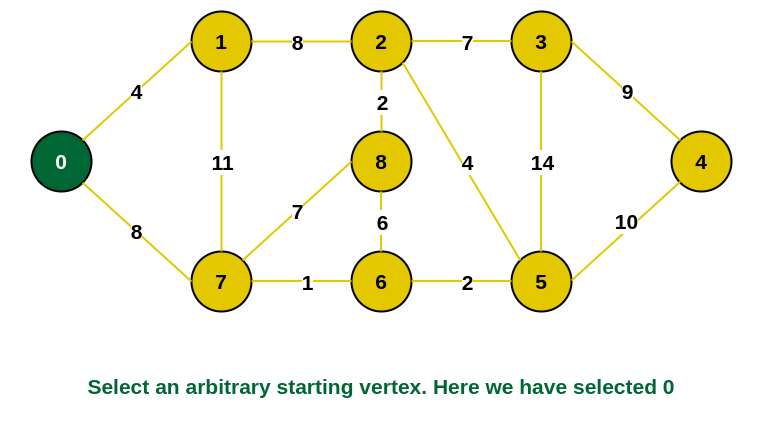0 is selected as starting vertex

Step 2: All the edges connecting the incomplete MST and other vertices are the edges {0, 1} and {0, 7}. Between these two the edge with minimum weight is {0, 1}. So include the edge and vertex 1 in the MST.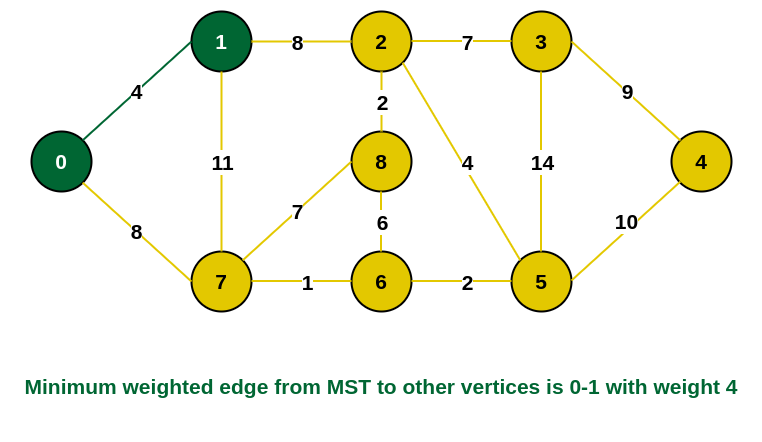1 is added to the MST

Step 3: The edges connecting the incomplete MST to other vertices are {0, 7}, {1, 7} and {1, 2}. Among these edges the minimum weight is 8 which is of the edges {0, 7} and {1, 2}. Let us here include the edge {0, 7} and the vertex 7 in the MST. [We could have also included edge {1, 2} and vertex 2 in the MST].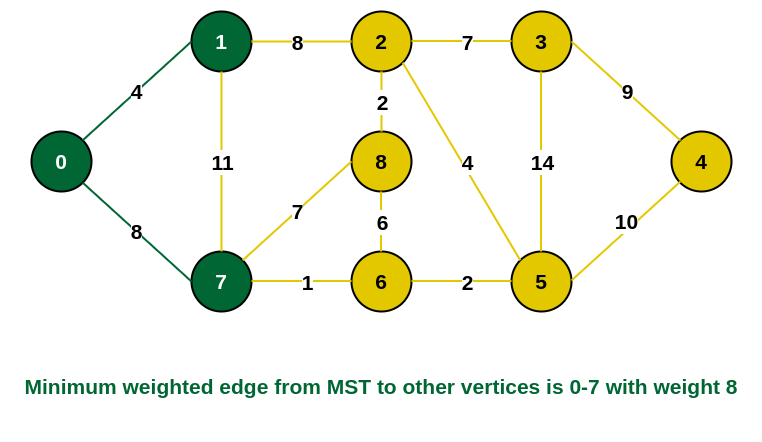7 is added in the MST

Step 4: The edges that connect the incomplete MST with the fringe vertices are {1, 2}, {7, 6} and {7, 8}. Add the edge {7, 6} and the vertex 6 in the MST as it has the least weight (i.e., 1).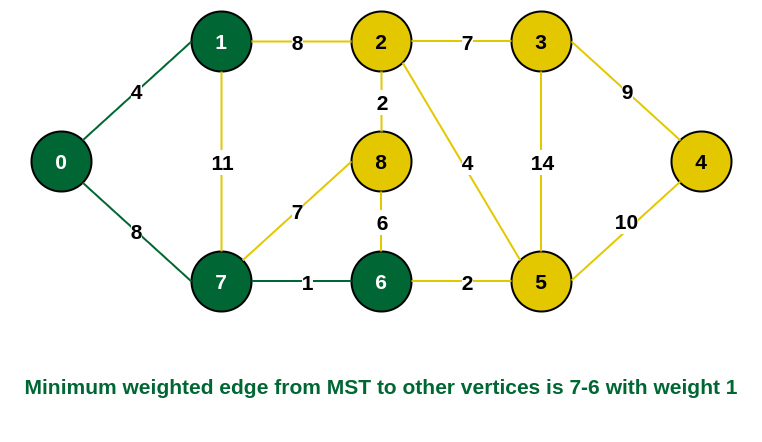6 is added in the MST

Step 5: The connecting edges now are {7, 8}, {1, 2}, {6, 8} and {6, 5}. Include edge {6, 5} and vertex 5 in the MST as the edge has the minimum weight (i.e., 2) among them.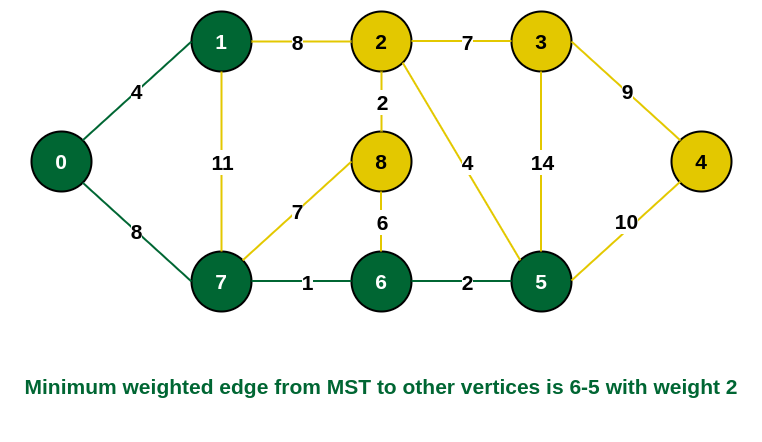Include vertex 5 in the MST

Step 6: Among the current connecting edges, the edge {5, 2} has the minimum weight. So include that edge and the vertex 2 in the MST.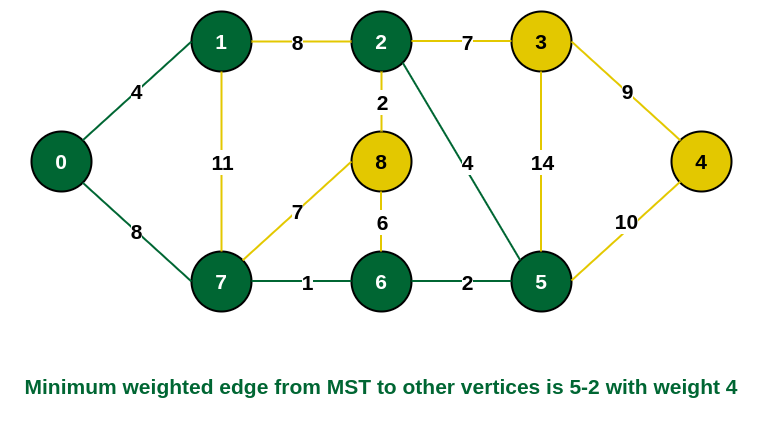Include vertex 2 in the MST

Step 7: The connecting edges between the incomplete MST and the other edges are {2, 8}, {2, 3}, {5, 3} and {5, 4}. The edge with minimum weight is edge {2, 8} which has weight 2. So include this edge and the vertex 8 in the MST.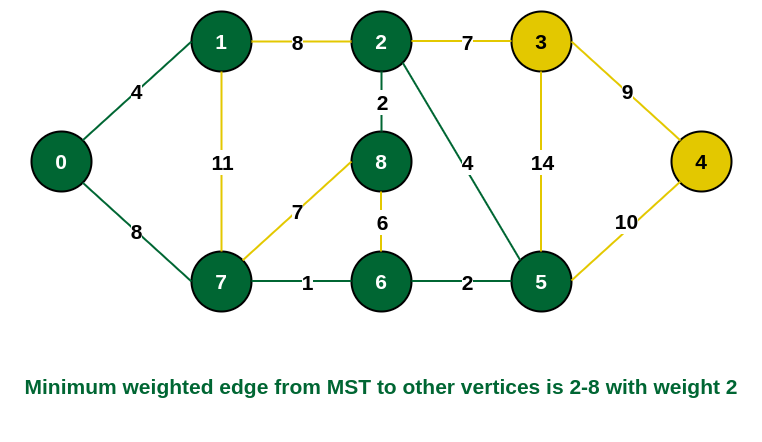Add vertex 8 in the MST

Step 8: See here that the edges {7, 8} and {2, 3} both have same weight which are minimum. But 7 is already part of MST. So we will consider the edge {2, 3} and include that edge and vertex 3 in the MST.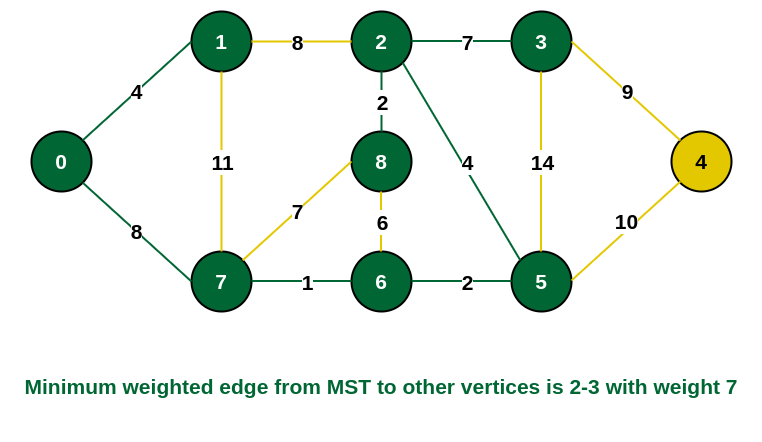Include vertex 3 in MST

Step 9: Only  the vertex 4 remains to be included. The minimum weighted edge from the incomplete MST to 4 is {3, 4}.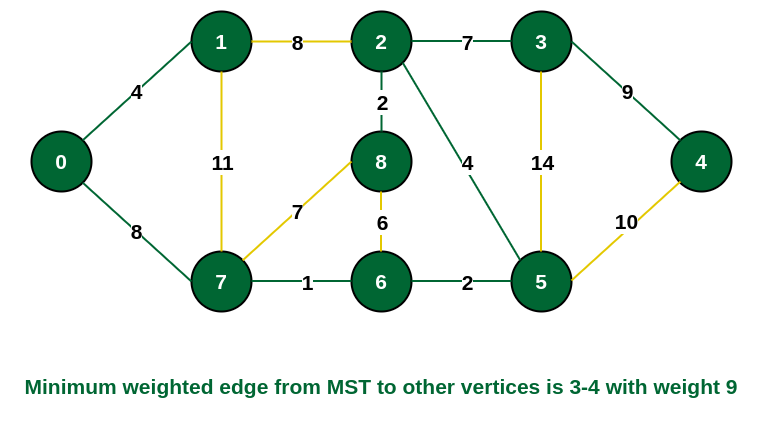Include vertex 4 in the MST

The final structure of the MST is as follows and the weight of the edges of the MST is (4 + 8 + 1 + 2 + 4 + 2 + 7 + 9) = 37.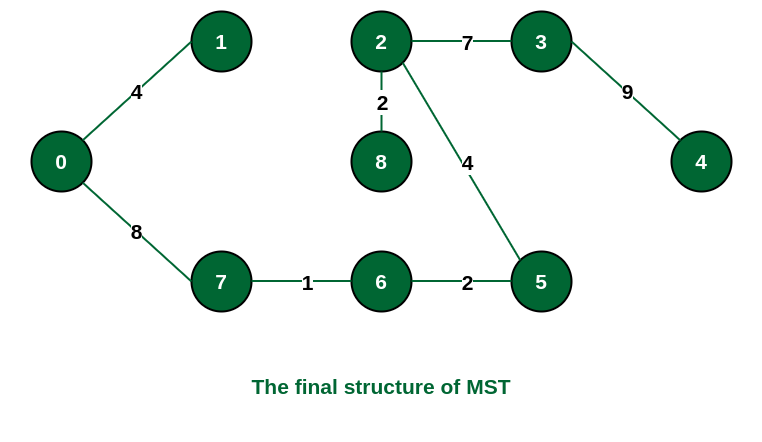The structure of the MST formed using the above method

Note: If we had selected the edge {1, 2} in the third step then the MST would look like the following.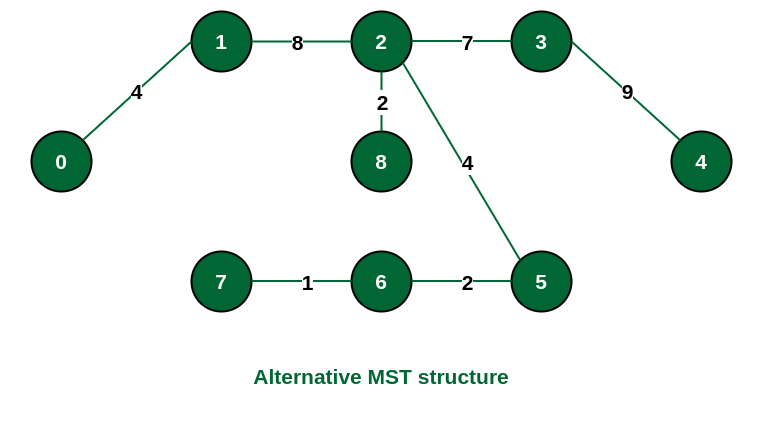Structure of the alternate MST if we had selected edge {1, 2} in the MST

### How to implement Prim’s Algorithm?

Follow the given steps to utilize the Prim’s Algorithm mentioned above for finding MST of a graph:

• Create a set mstSet that keeps track of vertices already included in MST.
• Assign a key value to all vertices in the input graph. Initialize all key values as INFINITE. Assign the key value as 0 for the first vertex so that it is picked first.
• While mstSet doesn’t include all vertices
• Pick a vertex u that is not there in mstSet and has a minimum key value.
• Include u in the mstSet
• Update the key value of all adjacent vertices of u. To update the key values, iterate through all adjacent vertices.
• For every adjacent vertex v, if the weight of edge u-v is less than the previous key value of v, update the key value as the weight of u-v.

The idea of using key values is to pick the minimum weight edge from the cut. The key values are used only for vertices that are not yet included in MST, the key value for these vertices indicates the minimum weight edges connecting them to the set of vertices included in MST.

Below is the implementation of the approach:

## C++

 `// A C++ program for Prim's Minimum``// Spanning Tree (MST) algorithm. The program is``// for adjacency matrix representation of the graph` `#include ``using` `namespace` `std;` `// Number of vertices in the graph``#define V 5` `// A utility function to find the vertex with``// minimum key value, from the set of vertices``// not yet included in MST``int` `minKey(``int` `key[], ``bool` `mstSet[])``{``    ``// Initialize min value``    ``int` `min = INT_MAX, min_index;` `    ``for` `(``int` `v = 0; v < V; v++)``        ``if` `(mstSet[v] == ``false` `&& key[v] < min)``            ``min = key[v], min_index = v;` `    ``return` `min_index;``}` `// A utility function to print the``// constructed MST stored in parent[]``void` `printMST(``int` `parent[], ``int` `graph[V][V])``{``    ``cout << ``"Edge \tWeight\n"``;``    ``for` `(``int` `i = 1; i < V; i++)``        ``cout << parent[i] << ``" - "` `<< i << ``" \t"``             ``<< graph[i][parent[i]] << ``" \n"``;``}` `// Function to construct and print MST for``// a graph represented using adjacency``// matrix representation``void` `primMST(``int` `graph[V][V])``{``    ``// Array to store constructed MST``    ``int` `parent[V];` `    ``// Key values used to pick minimum weight edge in cut``    ``int` `key[V];` `    ``// To represent set of vertices included in MST``    ``bool` `mstSet[V];` `    ``// Initialize all keys as INFINITE``    ``for` `(``int` `i = 0; i < V; i++)``        ``key[i] = INT_MAX, mstSet[i] = ``false``;` `    ``// Always include first 1st vertex in MST.``    ``// Make key 0 so that this vertex is picked as first``    ``// vertex.``    ``key = 0;``  ` `    ``// First node is always root of MST``    ``parent = -1;` `    ``// The MST will have V vertices``    ``for` `(``int` `count = 0; count < V - 1; count++) {``        ` `        ``// Pick the minimum key vertex from the``        ``// set of vertices not yet included in MST``        ``int` `u = minKey(key, mstSet);` `        ``// Add the picked vertex to the MST Set``        ``mstSet[u] = ``true``;` `        ``// Update key value and parent index of``        ``// the adjacent vertices of the picked vertex.``        ``// Consider only those vertices which are not``        ``// yet included in MST``        ``for` `(``int` `v = 0; v < V; v++)` `            ``// graph[u][v] is non zero only for adjacent``            ``// vertices of m mstSet[v] is false for vertices``            ``// not yet included in MST Update the key only``            ``// if graph[u][v] is smaller than key[v]``            ``if` `(graph[u][v] && mstSet[v] == ``false``                ``&& graph[u][v] < key[v])``                ``parent[v] = u, key[v] = graph[u][v];``    ``}` `    ``// Print the constructed MST``    ``printMST(parent, graph);``}` `// Driver's code``int` `main()``{``    ``int` `graph[V][V] = { { 0, 2, 0, 6, 0 },``                        ``{ 2, 0, 3, 8, 5 },``                        ``{ 0, 3, 0, 0, 7 },``                        ``{ 6, 8, 0, 0, 9 },``                        ``{ 0, 5, 7, 9, 0 } };` `    ``// Print the solution``    ``primMST(graph);` `    ``return` `0;``}` `// This code is contributed by rathbhupendra`

## C

 `// A C program for Prim's Minimum``// Spanning Tree (MST) algorithm. The program is``// for adjacency matrix representation of the graph` `#include ``#include ``#include ` `// Number of vertices in the graph``#define V 5` `// A utility function to find the vertex with``// minimum key value, from the set of vertices``// not yet included in MST``int` `minKey(``int` `key[], ``bool` `mstSet[])``{``    ``// Initialize min value``    ``int` `min = INT_MAX, min_index;` `    ``for` `(``int` `v = 0; v < V; v++)``        ``if` `(mstSet[v] == ``false` `&& key[v] < min)``            ``min = key[v], min_index = v;` `    ``return` `min_index;``}` `// A utility function to print the``// constructed MST stored in parent[]``int` `printMST(``int` `parent[], ``int` `graph[V][V])``{``    ``printf``(``"Edge \tWeight\n"``);``    ``for` `(``int` `i = 1; i < V; i++)``        ``printf``(``"%d - %d \t%d \n"``, parent[i], i,``               ``graph[i][parent[i]]);``}` `// Function to construct and print MST for``// a graph represented using adjacency``// matrix representation``void` `primMST(``int` `graph[V][V])``{``    ``// Array to store constructed MST``    ``int` `parent[V];``    ``// Key values used to pick minimum weight edge in cut``    ``int` `key[V];``    ``// To represent set of vertices included in MST``    ``bool` `mstSet[V];` `    ``// Initialize all keys as INFINITE``    ``for` `(``int` `i = 0; i < V; i++)``        ``key[i] = INT_MAX, mstSet[i] = ``false``;` `    ``// Always include first 1st vertex in MST.``    ``// Make key 0 so that this vertex is picked as first``    ``// vertex.``    ``key = 0;``  ` `    ``// First node is always root of MST``    ``parent = -1;` `    ``// The MST will have V vertices``    ``for` `(``int` `count = 0; count < V - 1; count++) {``        ` `        ``// Pick the minimum key vertex from the``        ``// set of vertices not yet included in MST``        ``int` `u = minKey(key, mstSet);` `        ``// Add the picked vertex to the MST Set``        ``mstSet[u] = ``true``;` `        ``// Update key value and parent index of``        ``// the adjacent vertices of the picked vertex.``        ``// Consider only those vertices which are not``        ``// yet included in MST``        ``for` `(``int` `v = 0; v < V; v++)` `            ``// graph[u][v] is non zero only for adjacent``            ``// vertices of m mstSet[v] is false for vertices``            ``// not yet included in MST Update the key only``            ``// if graph[u][v] is smaller than key[v]``            ``if` `(graph[u][v] && mstSet[v] == ``false``                ``&& graph[u][v] < key[v])``                ``parent[v] = u, key[v] = graph[u][v];``    ``}` `    ``// print the constructed MST``    ``printMST(parent, graph);``}` `// Driver's code``int` `main()``{``    ``int` `graph[V][V] = { { 0, 2, 0, 6, 0 },``                        ``{ 2, 0, 3, 8, 5 },``                        ``{ 0, 3, 0, 0, 7 },``                        ``{ 6, 8, 0, 0, 9 },``                        ``{ 0, 5, 7, 9, 0 } };` `    ``// Print the solution``    ``primMST(graph);` `    ``return` `0;``}`

## Java

 `// A Java program for Prim's Minimum Spanning Tree (MST)``// algorithm. The program is for adjacency matrix``// representation of the graph` `import` `java.io.*;``import` `java.lang.*;``import` `java.util.*;` `class` `MST {` `    ``// Number of vertices in the graph``    ``private` `static` `final` `int` `V = ``5``;` `    ``// A utility function to find the vertex with minimum``    ``// key value, from the set of vertices not yet included``    ``// in MST``    ``int` `minKey(``int` `key[], Boolean mstSet[])``    ``{``        ``// Initialize min value``        ``int` `min = Integer.MAX_VALUE, min_index = -``1``;` `        ``for` `(``int` `v = ``0``; v < V; v++)``            ``if` `(mstSet[v] == ``false` `&& key[v] < min) {``                ``min = key[v];``                ``min_index = v;``            ``}` `        ``return` `min_index;``    ``}` `    ``// A utility function to print the constructed MST``    ``// stored in parent[]``    ``void` `printMST(``int` `parent[], ``int` `graph[][])``    ``{``        ``System.out.println(``"Edge \tWeight"``);``        ``for` `(``int` `i = ``1``; i < V; i++)``            ``System.out.println(parent[i] + ``" - "` `+ i + ``"\t"``                               ``+ graph[i][parent[i]]);``    ``}` `    ``// Function to construct and print MST for a graph``    ``// represented using adjacency matrix representation``    ``void` `primMST(``int` `graph[][])``    ``{``        ``// Array to store constructed MST``        ``int` `parent[] = ``new` `int``[V];` `        ``// Key values used to pick minimum weight edge in``        ``// cut``        ``int` `key[] = ``new` `int``[V];` `        ``// To represent set of vertices included in MST``        ``Boolean mstSet[] = ``new` `Boolean[V];` `        ``// Initialize all keys as INFINITE``        ``for` `(``int` `i = ``0``; i < V; i++) {``            ``key[i] = Integer.MAX_VALUE;``            ``mstSet[i] = ``false``;``        ``}` `        ``// Always include first 1st vertex in MST.``        ``// Make key 0 so that this vertex is``        ``// picked as first vertex``        ``key[``0``] = ``0``;``      ` `        ``// First node is always root of MST``        ``parent[``0``] = -``1``;` `        ``// The MST will have V vertices``        ``for` `(``int` `count = ``0``; count < V - ``1``; count++) {``            ` `            ``// Pick the minimum key vertex from the set of``            ``// vertices not yet included in MST``            ``int` `u = minKey(key, mstSet);` `            ``// Add the picked vertex to the MST Set``            ``mstSet[u] = ``true``;` `            ``// Update key value and parent index of the``            ``// adjacent vertices of the picked vertex.``            ``// Consider only those vertices which are not``            ``// yet included in MST``            ``for` `(``int` `v = ``0``; v < V; v++)` `                ``// graph[u][v] is non zero only for adjacent``                ``// vertices of m mstSet[v] is false for``                ``// vertices not yet included in MST Update``                ``// the key only if graph[u][v] is smaller``                ``// than key[v]``                ``if` `(graph[u][v] != ``0` `&& mstSet[v] == ``false``                    ``&& graph[u][v] < key[v]) {``                    ``parent[v] = u;``                    ``key[v] = graph[u][v];``                ``}``        ``}` `        ``// Print the constructed MST``        ``printMST(parent, graph);``    ``}` `    ``public` `static` `void` `main(String[] args)``    ``{``        ``MST t = ``new` `MST();``        ``int` `graph[][] = ``new` `int``[][] { { ``0``, ``2``, ``0``, ``6``, ``0` `},``                                      ``{ ``2``, ``0``, ``3``, ``8``, ``5` `},``                                      ``{ ``0``, ``3``, ``0``, ``0``, ``7` `},``                                      ``{ ``6``, ``8``, ``0``, ``0``, ``9` `},``                                      ``{ ``0``, ``5``, ``7``, ``9``, ``0` `} };` `        ``// Print the solution``        ``t.primMST(graph);``    ``}``}` `// This code is contributed by Aakash Hasija`

## Python3

 `# A Python3 program for``# Prim's Minimum Spanning Tree (MST) algorithm.``# The program is for adjacency matrix``# representation of the graph` `# Library for INT_MAX``import` `sys`  `class` `Graph():``    ``def` `__init__(``self``, vertices):``        ``self``.V ``=` `vertices``        ``self``.graph ``=` `[[``0` `for` `column ``in` `range``(vertices)]``                      ``for` `row ``in` `range``(vertices)]` `    ``# A utility function to print``    ``# the constructed MST stored in parent[]``    ``def` `printMST(``self``, parent):``        ``print``(``"Edge \tWeight"``)``        ``for` `i ``in` `range``(``1``, ``self``.V):``            ``print``(parent[i], ``"-"``, i, ``"\t"``, ``self``.graph[i][parent[i]])` `    ``# A utility function to find the vertex with``    ``# minimum distance value, from the set of vertices``    ``# not yet included in shortest path tree``    ``def` `minKey(``self``, key, mstSet):` `        ``# Initialize min value``        ``min` `=` `sys.maxsize` `        ``for` `v ``in` `range``(``self``.V):``            ``if` `key[v] < ``min` `and` `mstSet[v] ``=``=` `False``:``                ``min` `=` `key[v]``                ``min_index ``=` `v` `        ``return` `min_index` `    ``# Function to construct and print MST for a graph``    ``# represented using adjacency matrix representation``    ``def` `primMST(``self``):` `        ``# Key values used to pick minimum weight edge in cut``        ``key ``=` `[sys.maxsize] ``*` `self``.V``        ``parent ``=` `[``None``] ``*` `self``.V  ``# Array to store constructed MST``        ``# Make key 0 so that this vertex is picked as first vertex``        ``key[``0``] ``=` `0``        ``mstSet ``=` `[``False``] ``*` `self``.V` `        ``parent[``0``] ``=` `-``1`  `# First node is always the root of` `        ``for` `cout ``in` `range``(``self``.V):` `            ``# Pick the minimum distance vertex from``            ``# the set of vertices not yet processed.``            ``# u is always equal to src in first iteration``            ``u ``=` `self``.minKey(key, mstSet)` `            ``# Put the minimum distance vertex in``            ``# the shortest path tree``            ``mstSet[u] ``=` `True` `            ``# Update dist value of the adjacent vertices``            ``# of the picked vertex only if the current``            ``# distance is greater than new distance and``            ``# the vertex in not in the shortest path tree``            ``for` `v ``in` `range``(``self``.V):` `                ``# graph[u][v] is non zero only for adjacent vertices of m``                ``# mstSet[v] is false for vertices not yet included in MST``                ``# Update the key only if graph[u][v] is smaller than key[v]``                ``if` `self``.graph[u][v] > ``0` `and` `mstSet[v] ``=``=` `False` `\``                ``and` `key[v] > ``self``.graph[u][v]:``                    ``key[v] ``=` `self``.graph[u][v]``                    ``parent[v] ``=` `u` `        ``self``.printMST(parent)`  `# Driver's code``if` `__name__ ``=``=` `'__main__'``:``    ``g ``=` `Graph(``5``)``    ``g.graph ``=` `[[``0``, ``2``, ``0``, ``6``, ``0``],``               ``[``2``, ``0``, ``3``, ``8``, ``5``],``               ``[``0``, ``3``, ``0``, ``0``, ``7``],``               ``[``6``, ``8``, ``0``, ``0``, ``9``],``               ``[``0``, ``5``, ``7``, ``9``, ``0``]]` `    ``g.primMST()`  `# Contributed by Divyanshu Mehta`

## C#

 `// A C# program for Prim's Minimum``// Spanning Tree (MST) algorithm.``// The program is for adjacency``// matrix representation of the graph` `using` `System;``class` `MST {` `    ``// Number of vertices in the graph``    ``static` `int` `V = 5;` `    ``// A utility function to find``    ``// the vertex with minimum key``    ``// value, from the set of vertices``    ``// not yet included in MST``    ``static` `int` `minKey(``int``[] key, ``bool``[] mstSet)``    ``{``        ``// Initialize min value``        ``int` `min = ``int``.MaxValue, min_index = -1;` `        ``for` `(``int` `v = 0; v < V; v++)``            ``if` `(mstSet[v] == ``false` `&& key[v] < min) {``                ``min = key[v];``                ``min_index = v;``            ``}` `        ``return` `min_index;``    ``}` `    ``// A utility function to print``    ``// the constructed MST stored in parent[]``    ``static` `void` `printMST(``int``[] parent, ``int``[, ] graph)``    ``{``        ``Console.WriteLine(``"Edge \tWeight"``);``        ``for` `(``int` `i = 1; i < V; i++)``            ``Console.WriteLine(parent[i] + ``" - "` `+ i + ``"\t"``                              ``+ graph[i, parent[i]]);``    ``}` `    ``// Function to construct and``    ``// print MST for a graph represented``    ``// using adjacency matrix representation``    ``static` `void` `primMST(``int``[, ] graph)``    ``{``        ``// Array to store constructed MST``        ``int``[] parent = ``new` `int``[V];` `        ``// Key values used to pick``        ``// minimum weight edge in cut``        ``int``[] key = ``new` `int``[V];` `        ``// To represent set of vertices``        ``// included in MST``        ``bool``[] mstSet = ``new` `bool``[V];` `        ``// Initialize all keys``        ``// as INFINITE``        ``for` `(``int` `i = 0; i < V; i++) {``            ``key[i] = ``int``.MaxValue;``            ``mstSet[i] = ``false``;``        ``}` `        ``// Always include first 1st vertex in MST.``        ``// Make key 0 so that this vertex is``        ``// picked as first vertex``        ``// First node is always root of MST``        ``key = 0;``        ``parent = -1;` `        ``// The MST will have V vertices``        ``for` `(``int` `count = 0; count < V - 1; count++) {` `            ``// Pick the minimum key vertex``            ``// from the set of vertices``            ``// not yet included in MST``            ``int` `u = minKey(key, mstSet);` `            ``// Add the picked vertex``            ``// to the MST Set``            ``mstSet[u] = ``true``;` `            ``// Update key value and parent``            ``// index of the adjacent vertices``            ``// of the picked vertex. Consider``            ``// only those vertices which are``            ``// not yet included in MST``            ``for` `(``int` `v = 0; v < V; v++)` `                ``// graph[u][v] is non zero only``                ``// for adjacent vertices of m``                ``// mstSet[v] is false for vertices``                ``// not yet included in MST Update``                ``// the key only if graph[u][v] is``                ``// smaller than key[v]``                ``if` `(graph[u, v] != 0 && mstSet[v] == ``false``                    ``&& graph[u, v] < key[v]) {``                    ``parent[v] = u;``                    ``key[v] = graph[u, v];``                ``}``        ``}` `        ``// Print the constructed MST``        ``printMST(parent, graph);``    ``}` `    ``// Driver's Code``    ``public` `static` `void` `Main()``    ``{``        ``int``[, ] graph = ``new` `int``[, ] { { 0, 2, 0, 6, 0 },``                                      ``{ 2, 0, 3, 8, 5 },``                                      ``{ 0, 3, 0, 0, 7 },``                                      ``{ 6, 8, 0, 0, 9 },``                                      ``{ 0, 5, 7, 9, 0 } };` `        ``// Print the solution``        ``primMST(graph);``    ``}``}` `// This code is contributed by anuj_67.`

## Javascript

 ``

Output

```Edge     Weight
0 - 1     2
1 - 2     3
0 - 3     6
1 - 4     5

```

Time Complexity: O(V2), If the input graph is represented using an adjacency list, then the time complexity of Prim’s algorithm can be reduced to O(E * logV) with the help of a binary heap.  In this implementation, we are always considering the spanning tree to start from the root of the graph
Auxiliary Space: O(V)

### Other Implementations of Prim’s Algorithm:

Given below are some other implementations of Prim’s Algorithm

#### OPTIMIZED APPROACH OF PRIM’S ALGORITHM:

Intuition

2. Then we create a Pair class to store the vertex and its weight .
3. We sort the list on the basis of lowest weight.
4. We create priority queue and push the first vertex and its weight in the queue
5. Then we just traverse through its edges and store the least weight in a variable called ans.
6. At last after all the vertex we return the ans.

Implementation

## C++

 `#include``using` `namespace` `std;` `typedef` `pair<``int``,``int``> pii;` `// Function to find sum of weights of edges of the Minimum Spanning Tree.``int` `spanningTree(``int` `V, ``int` `E, ``int` `edges[])``{  ``    ``// Create an adjacency list representation of the graph``    ``vector> adj[V];``    ` `    ``// Fill the adjacency list with edges and their weights``    ``for` `(``int` `i = 0; i < E; i++) {``        ``int` `u = edges[i];``        ``int` `v = edges[i];``        ``int` `wt = edges[i];``        ``adj[u].push_back({v, wt});``        ``adj[v].push_back({u, wt});``    ``}``    ` `    ``// Create a priority queue to store edges with their weights``    ``priority_queue, greater> pq;``    ` `    ``// Create a visited array to keep track of visited vertices``    ``vector<``bool``> visited(V, ``false``);``    ` `    ``// Variable to store the result (sum of edge weights)``    ``int` `res = 0;``    ` `    ``// Start with vertex 0``    ``pq.push({0, 0});``    ` `    ``// Perform Prim's algorithm to find the Minimum Spanning Tree``    ``while``(!pq.empty()){``        ``auto` `p = pq.top();``        ``pq.pop();``        ` `        ``int` `wt = p.first;  ``// Weight of the edge``        ``int` `u = p.second;  ``// Vertex connected to the edge``        ` `        ``if``(visited[u] == ``true``){``            ``continue``;  ``// Skip if the vertex is already visited``        ``}``        ` `        ``res += wt;  ``// Add the edge weight to the result``        ``visited[u] = ``true``;  ``// Mark the vertex as visited``        ` `        ``// Explore the adjacent vertices``        ``for``(``auto` `v : adj[u]){``            ``// v represents the vertex and v represents the edge weight``            ``if``(visited[v] == ``false``){``                ``pq.push({v, v});  ``// Add the adjacent edge to the priority queue``            ``}``        ``}``    ``}``    ` `    ``return` `res;  ``// Return the sum of edge weights of the Minimum Spanning Tree``}` `int` `main()``{``    ``int` `graph[] = {{0, 1, 5},``                      ``{1, 2, 3},``                      ``{0, 2, 1}};` `    ``// Function call``    ``cout << spanningTree(3, 3, graph) << endl;` `    ``return` `0;``}`

## Java

 `// A Java program for Prim's Minimum Spanning Tree (MST)``// algorithm. The program is for adjacency list``// representation of the graph` `import` `java.io.*;``import` `java.util.*;` `// Class to form pair``class` `Pair ``implements` `Comparable``{``    ``int` `v;``    ``int` `wt;``    ``Pair(``int` `v,``int` `wt)``    ``{``        ``this``.v=v;``        ``this``.wt=wt;``    ``}``    ``public` `int` `compareTo(Pair that)``    ``{``        ``return` `this``.wt-that.wt;``    ``}``}` `class` `GFG {` `// Function of spanning tree``static` `int` `spanningTree(``int` `V, ``int` `E, ``int` `edges[][])``    ``{``         ``ArrayList> adj=``new` `ArrayList<>();``         ``for``(``int` `i=``0``;i());``         ``}``         ``for``(``int` `i=``0``;i pq = ``new` `PriorityQueue();``         ``pq.add(``new` `Pair(``0``,``0``));``         ``int``[] vis=``new` `int``[V];``         ``int` `s=``0``;``         ``while``(!pq.isEmpty())``         ``{``             ``Pair node=pq.poll();``             ``int` `v=node.v;``             ``int` `wt=node.wt;``             ``if``(vis[v]==``1``)``             ``continue``;``             ` `             ``s+=wt;``             ``vis[v]=``1``;``             ``for``(Pair it:adj.get(v))``             ``{``                 ``if``(vis[it.v]==``0``)``                 ``{``                     ``pq.add(``new` `Pair(it.v,it.wt));``                 ``}``             ``}``         ``}``         ``return` `s;``    ``}``    ` `    ``// Driver code``    ``public` `static` `void` `main (String[] args) {``        ``int` `graph[][] = ``new` `int``[][] {{``0``,``1``,``5``},``                                    ``{``1``,``2``,``3``},``                                    ``{``0``,``2``,``1``}};`` ` `        ``// Function call``        ``System.out.println(spanningTree(``3``,``3``,graph));``    ``}``}`

## Python3

 `import` `heapq` `def` `tree(V, E, edges):``    ``# Create an adjacency list representation of the graph``    ``adj ``=` `[[] ``for` `_ ``in` `range``(V)]``    ``# Fill the adjacency list with edges and their weights``    ``for` `i ``in` `range``(E):``        ``u, v, wt ``=` `edges[i]``        ``adj[u].append((v, wt))``        ``adj[v].append((u, wt))``    ``# Create a priority queue to store edges with their weights``    ``pq ``=` `[]``    ``# Create a visited array to keep track of visited vertices``    ``visited ``=` `[``False``] ``*` `V``    ``# Variable to store the result (sum of edge weights)``    ``res ``=` `0``    ``# Start with vertex 0``    ``heapq.heappush(pq, (``0``, ``0``))``    ``# Perform Prim's algorithm to find the Minimum Spanning Tree``    ``while` `pq:``        ``wt, u ``=` `heapq.heappop(pq)``        ``if` `visited[u]:``            ``continue` `            ``# Skip if the vertex is already visited``        ``res ``+``=` `wt ``        ``# Add the edge weight to the result``        ``visited[u] ``=` `True` `        ``# Mark the vertex as visited``        ``# Explore the adjacent vertices``        ``for` `v, weight ``in` `adj[u]:``            ``if` `not` `visited[v]:``                ``heapq.heappush(pq, (weight, v)) ``                ``# Add the adjacent edge to the priority queue``    ``return` `res ``  ``# Return the sum of edge weights of the Minimum Spanning Tree``if` `__name__ ``=``=` `"__main__"``:``    ``graph ``=` `[[``0``, ``1``, ``5``],``             ``[``1``, ``2``, ``3``],``             ``[``0``, ``2``, ``1``]]``    ``# Function call``    ``print``(tree(``3``, ``3``, graph))`

Output

```4

```

Time Complexity: O(E*log(E)) where E is the number of edges
Auxiliary Space: O(V^2) where V is the number of vertex